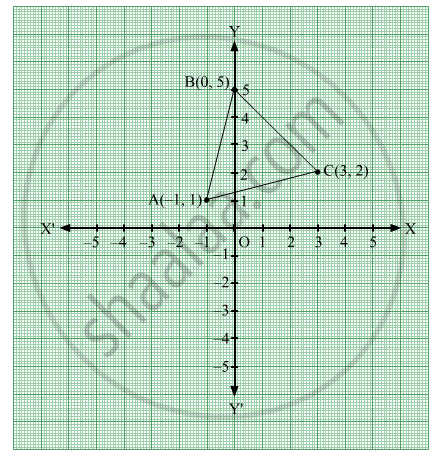# Find the Area of the Triangle Whose Vertices Are (-1, 1), (0, 5) and (3, 2), Using Integration. - Mathematics

Graph
Sum

Find the area of the triangle whose vertices are (-1, 1), (0, 5) and (3, 2), using integration.

#### SolutionLet A (-1,1), B (0,5) and C (3,2)

The equation of line AB is

y -1 = (5 -1)/(0+ 1) ("x"+1)

y = 4"x" + 5

The equation of line BC is

y - 5 = (2 -5)/(3 -0) ("x" -0)

y = -"x"+5

The equation of line CA is

y - 2 = (1 -2)/(-1 -3) ("x" -3)

y = ("x")/(4) + (5)/(4)

Required area = Area of ΔABC

The equation of line CA is

y - 2 = (1 -2)/(-1 -3) ("x" -3)

y = ("x")/(4) + (5)/(4)

Required area = Area of ΔABC
= int_-1^0 (4x + 5) dx + int_0^3 (- x + 5) dx - int_-1^3 ( x/4 + 5/4) dx

= [ 2x^2 + 5x ]_-1^0 + [ -x^2/2 + 5x ]_0^3 - [ x^2/8 + 5x/4 ]_-1^3

= 3 + 21/2 - 39/8 - 9/8

= 15/2 sq. units

Concept: Integration Using Trigonometric Identities
Is there an error in this question or solution?